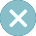# Formula (calculated) columns

## Formula (calculated) columns in wpDataTablesHow to create and use formula-based columns

If your dataset used for wpDataTable creation is not comprehensive – if for example, it shows only the price without VAT tax, or you need a column showing a result of calculation based on other columns’ cell values, you can use the formula (calculated) columns.

See this table: “Tax” and “Difference” columns do not exist in the dataset. They are calculated “on the fly” using the formula columns feature.

Please note that formulas in wpDataTables are NOT THE SAME as formulas in Excel!

## Add a formula columnCreating a formula column

Any table can have a formula column. To add one, you can click the “Add a formula column” button:

## Formula constructorBuilding a formula and testing it

After you click the “Add formula a column” button, you will see a popup formula constructor. It has a fixed name ‘formula_1’, ‘formula_2’, etc. – depending on the amount of formulas that you added.

The formula constructor popup has several main elements:

1. Columns that can be used in the formula. Please note that only numeric (float and integer columns) can be used in formula columns.
2. Formula. The created formula itself.
3. Supported operators. Formulas in wpDataTables support all arithmetic operators, brackets to define the calculation order, and trigonometric operators.
4. Preview button and preview section. Once you prepare the formula, you can click this button to see the result for the first 5 rows of your table to verify the calculation is correct.

Additionally, there is an explanation text on the top.

You can use columns (values for each cell will be inserted), or number values. Only numeric columns are allowed (non-numeric will be parsed as 0). Basic math operations and brackets are supported. Example: col1*((col2+2)-col3*sin(col4-3)).

To calculate the “Tax” column in the above example, we can simply use the catalog cost column value, multiply it by some number, and click “Save“.

Now let’s change the displayed header of the formula column. To do that, click the button for column settings.

Enter the new value in the “Displayed header” input in the first tab column setting and click apply.

If you want to change the formula for a calculation you can go to the “Data” tab and click on the “Open Formula Editor” button.
After you click on THE “Open Formula Editor” button, the formula constructor popup will appear. You can use it to adjust the formula that is used for calculation.

Formula columns can be easily deleted by clicking the “Delete” button in the column header area in the live table preview.

There are several limitations when using formula columns in wpDataTables:

1. One formula column cannot be used in another. This limitation can be avoided by using nested calculations.
2. In tables with server-side processing formula, columns cannot be used for grouping, filtering, and sorting. Basically, formulas are calculated only for the rows that are immediately visible; Therefore, it’s not possible to “know” the values for currently invisible rows. Please note that this also applies to the “Manual” tables, as they also use server-side processing by default. Also, of course, it is not possible to edit the cells generated by formulas in the editable tables.
3. Adding a sum in the sum row for formula columns is not yet supported.
4. Using calculation functions in formula columns is not possibleDo you have the best

essential plugins installed?

Grab the best 46 plugins. Tested and sorted.

Plugins for SEO, Caching, Security, Design and UX, Stats & analytics, Social sharing. Only the best ones.

By clicking "subscribe" you consent that the information you provide via this form will be securely processed and stored in compliance with EU privacy laws (GDPR).